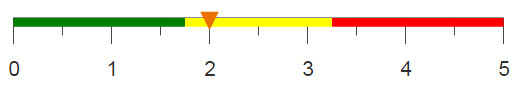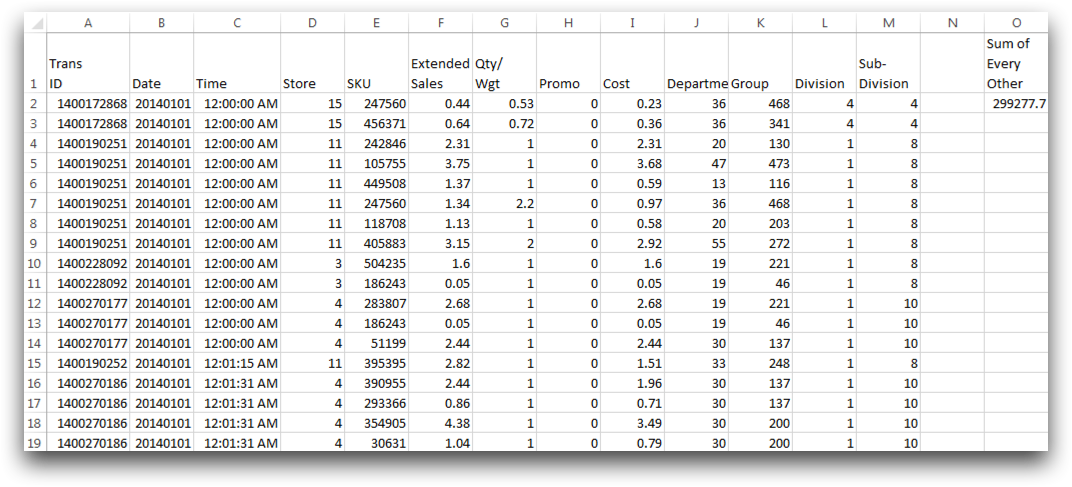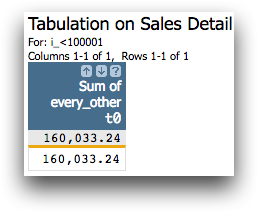# Summing every other value

Using the mod function makes it simple to sum every other value both in Excel and 1010data.

## Difficulty## Objective

You would like to compute the sum of every other entry in a table. You know how to do this in Excel by using a combinations of the functions SUM, IF, MOD, ROW, INDIRECT, and COUNT, and you would like to perform the same operations using 1010data.

## Excel solution

Using the functions SUM, IF, MOD, ROW, INDIRECT, and COUNT, Excel is able to divide the row numbers by 2 in order to determine if that row should be included in the sum, and then sum the selected values using the equation below.

`{=SUM(IF(MOD(ROW(INDIRECT("1:"&COUNT(F2:F100001)))-1,2)=0,F2:F100001,""))}`

The worksheet including the solution, can be seen in the image below.However, Excel cannot process the entire Sales Detail table because it is too large. Therefore, these operations are only done on a subset of the table containing 100,000 rows. 1010data on the other hand can perform these operations on the entire table at a much faster speed.

## 1010data Macro Language solution

```<base table="pub.doc.retail.altseg.sales_detail_transid"/>
<sel value="i_<100001"/>
<willbe name="every_other" value="if(mod(i_();2);xsales;0)"/>
<tabu label="Tabulation on Sales Detail">
<tcol source="every_other" fun="sum" label="Sum of`every_other"/>
</tabu>```

The selection done in the second line of this solution is used in order to produce the same results as the Excel solution, since Excel cannot process the table in its entirety. However, 1010data can perform the same operations on the entire table, without any additional time constraint.

To sum every other row, first a column called every_other, is created using the <willbe> operation. Within the value attribute of this column, the function mod(X;Y) is used to determine which rows are even and which are odd by returning a 0 if the row number is divisible by 2 and 1 if it is not divisible by 2. i_ is the system variable for row number, and in this case, the sticky version,i_(), is used so that the row numbers don't change as the table changes. Then the if function fills the column with the extended sales value if mod returns 1, and 0 otherwise.

After this column is created, you can then perform a tabulation to determine the sum of every other row. Within the <tcol> operation, the source column is every_other, and the function is fun="sum". The final table can be seen in the image below.## Further reading

If you would like to learn more about the functions and operations discussed in this recipe, click on the links below:

mod(X;Y)

<tabu>